# KS1 Maths Quiz - Year 2 Shapes - Describing 3D Shapes (Questions)

This quiz addresses the requirements of the National Curriculum KS1 Maths and Numeracy for children aged 6 and 7 in year 2. Specifically this quiz is aimed at the section dealing with naming and describing 3D shapes and related everyday objects fluently.

When talking about and naming 3-dimensional shapes and other related objects, Year 2 children will understand that they can use different words to describe their properties. They will use language such as faces, edges and corners and recognise that some shapes have the same name but may look different.

This quiz will familiarise your child with the language used to describe and to name 3-D shapes.

To see a larger image, click on the picture.
1. Which shapes can you see here?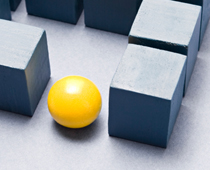[ ] Some cubes and a pyramid [ ] A cylinder and some pyramids [ ] A cone and a sphere [ ] Some cubes and a sphere
2. What shapes can you see here?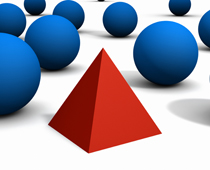[ ] Some cylinders and a cuboid [ ] Some spheres and a pyramid [ ] Some cuboids and a cone [ ] Some cones and a pyramid
3. Which of these shapes has the most edges?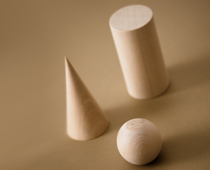[ ] The cone [ ] The cylinder [ ] The sphere [ ] They all have the same number of edges
4. This is a...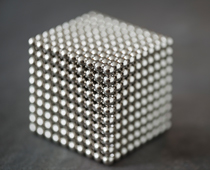[ ] cone made up of cylinders [ ] pyramid made up of cubes [ ] sphere made up of cubes [ ] cube made up of spheres
5. Which of these shapes has the most faces?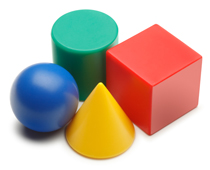[ ] The cone [ ] The cube [ ] The sphere [ ] The cylinder
6. How many corners does a cube have?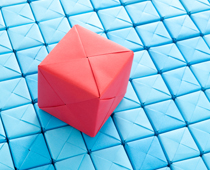[ ] 2 [ ] 4 [ ] 6 [ ] 8
7. Why are boxes usually cubes and cuboids?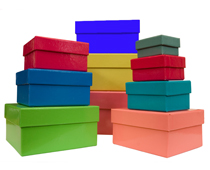[ ] They look good in shops [ ] They stack together well [ ] They have too many corners [ ] They can be big or small
8. Why do cubes make good dice?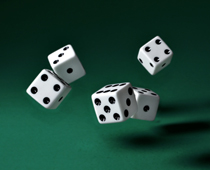[ ] They can roll but land flat [ ] They look better [ ] They have enough space for the dots [ ] They have enough faces
9. Why are snooker and pool balls spheres?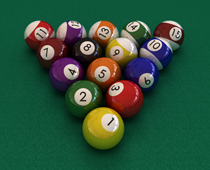[ ] Because they have numbers on [ ] Because they are a good size [ ] Because they look colourful [ ] Because they roll smoothly
10. If I try to fit the cuboid through the hole, what will happen?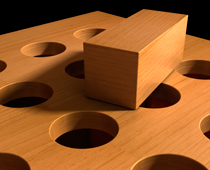[ ] If you twist it first, it will fit [ ] If you push it hard it will fit [ ] It is the wrong shape and will not fit [ ] It is the same shape as the hole so it will fitKS1 Maths Quiz - Year 2 Shapes - Describing 3D Shapes (Answers)
1. Which shapes can you see here?[ ] Some cubes and a pyramid [ ] A cylinder and some pyramids [ ] A cone and a sphere [x] Some cubes and a sphere
The cubes are also called cuboids
2. What shapes can you see here?[ ] Some cylinders and a cuboid [x] Some spheres and a pyramid [ ] Some cuboids and a cone [ ] Some cones and a pyramid
Pyramids can have square bases or triangular bases
3. Which of these shapes has the most edges?[ ] The cone [x] The cylinder [ ] The sphere [ ] They all have the same number of edges
The cylinder has two edges, the cone has one and the sphere has none at all
4. This is a...[ ] cone made up of cylinders [ ] pyramid made up of cubes [ ] sphere made up of cubes [x] cube made up of spheres
The spheres are arranged in even rows in order to create a cube shape
5. Which of these shapes has the most faces?[ ] The cone [x] The cube [ ] The sphere [ ] The cylinder
The cube has 6 faces, the cylinder has three, the cone has two and the sphere has one
6. How many corners does a cube have?[ ] 2 [ ] 4 [ ] 6 [x] 8
Corners are where the edges meet and can also be called vertices
7. Why are boxes usually cubes and cuboids?[ ] They look good in shops [x] They stack together well [ ] They have too many corners [ ] They can be big or small
Lots of cubes or cuboids stack together more easily than cylinders or cones
8. Why do cubes make good dice?[x] They can roll but land flat [ ] They look better [ ] They have enough space for the dots [ ] They have enough faces
A cube usually lands well so that one face is facing upwards
9. Why are snooker and pool balls spheres?[ ] Because they have numbers on [ ] Because they are a good size [ ] Because they look colourful [x] Because they roll smoothly
A perfect sphere rolls well - lots of sports have balls which are spheres
10. If I try to fit the cuboid through the hole, what will happen?[ ] If you twist it first, it will fit [ ] If you push it hard it will fit [x] It is the wrong shape and will not fit [ ] It is the same shape as the hole so it will fit
The edges of the cuboid will not fit through the round hole